Function Repository Resource:

# Uncurry

Reverse a Curry operation

Contributed by: Nikolay Murzin
 ResourceFunction["Uncurry"][f,n] represents an operator form of the function f of n arguments so that ResourceFunction["Uncurry"][f,n][x1,…,xn] is equivalent to f[x1]…[xn]. ResourceFunction["Uncurry"][f] represents an operator form of the function f of two arguments so that ResourceFunction["Uncurry"][f][x1,x2] is equivalent to f[x2][x1]. ResourceFunction["Uncurry"][f,{i1,…,in}] represents an operator form of the function f of n arguments so that ResourceFunction["Uncurry"][f,{i1,…,in}][x1,…,xn] is equivalent to f[xj1]…[xjn], where jk is the position of k in the permutation list {i1,…,in}. ResourceFunction["Uncurry"][f,k→{i1,…,in}] represents a k-arguments operator form of the function f of n arguments so that ResourceFunction["Uncurry"][f,k→{i1,…,in}][x1,…,xn] is equivalent to f[xj1]…[xjk], with k≥Max[{i1,…,in}], where jk is the position of k in the permutation list {i1,…,ik} with missing im filled in sequentially to form a complete permutation of length k.

## Details and Options

{j1,,jk} is equal to InversePermutation[{i1,,in}].

## Examples

### Basic Examples (1)

Uncurry a curried function:

 In:=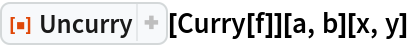Out=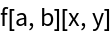In:=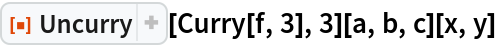Out=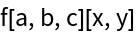In:=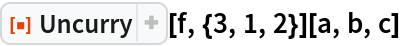Out=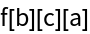In:=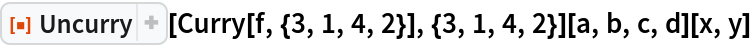Out=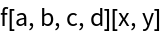In:=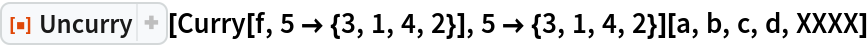Out=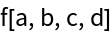### Possible Issues (2)

A curried function that ignores its arguments cannot be completely uncurried:

 In:=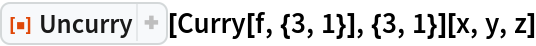Out=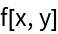The function loses an argument depending on its position in the uncurried permutation list:

 In:=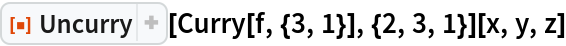Out=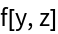In:=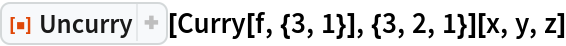Out=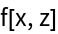In:=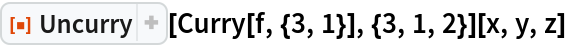Out=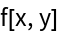N. Murzin

## Version History

• 1.0.0 – 02 March 2020